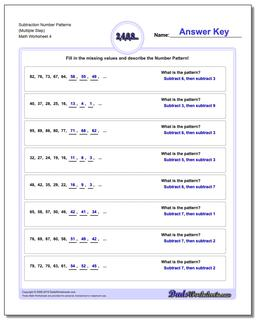# Math Worksheets: Number Patterns: Number Patterns: Subtraction Number Patterns (Multiple Step) (Fourth Worksheet)## Subtraction Number Patterns (Multiple Step) (Fourth Worksheet)

PropertyValue
DescriptionSubtraction Number Patterns (Multiple Step): Number pattern problems using only subtraction operations. (Fourth Worksheet)
Resource TypeWorksheet